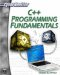# Function Basics

What are functions? In simple terms, a function is a block of code that is identified by some name and can take zero or more parameters and can return some value. That definition probably does little to clarify the issue for you! Let’s look at a function and its parts to help clarify this issue. To begin with, consider the following function, which squares a number.

`float square(float num) {   float answer = num * num;   return answer; }`

The first line of the function is called its declaration line. It has three parts. The first part is its return type. The return type tells you what kind of data the function will give you back. If it returns nothing, then its return type would be void. In the previous example, the function will return the answer to our math problem, and that is a float. The second thing is the name of the function. Function names follow the same guidelines as variable names. It is a good idea to have the function name reflect what the function will do. Next, inside the parenthesis, we have parameters. A parameter is some value you pass to the function in order for it to work. For example, in this case, to square a number, we have to give it the number to square. Students often ask: “What do we need to put as parameters? Do we even need any parameters for this function?” There is a simple way to answer this question for any function you write. Ask yourself this question: If you wanted some person to do this task for you, would you need to give them anything? And, if so, what? If you wanted a person to square a number for you, you would have to give them that number. However, if you just wanted them to say hello, you would not have to give them anything. Therefore, a function that squares a number should take one parameter, and a function that displays hello on the screen might not take any parameters. There will be a lot more in-depth discussion on functions in Chapter 4.C++ Programming Fundamentals (Cyberrookies)
ISBN: 1584502371
EAN: 2147483647
Year: 2005
Pages: 197
Authors: Chuck Easttom